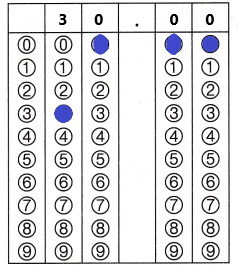Refer to our Texas Go Math Grade 5 Answer Key Pdf to score good marks in the exams. Test yourself by practicing the problems from Texas Go Math Grade 5 Module 8 Assessment Answer Key.

Concepts and Skills

Question 1.
Which value of n makes the equation, n + 5 = 4 + 4, true? (TEKS 5.4.B )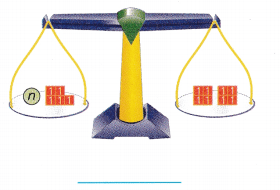n + 5 = 4 + 4
n + 5 = 8
3 + 5 = 8
The value n = 3 makes the equation true.
Explanation:
In the above pans balance. The Right side pan have 8 cubes and in the left side pan have n + 5 cubes. To get the balanced pans we need to add 3 cubes to the left side pan. So the value n = 3 makes the equation true.

Use the strip diagram to write an equation. Then solve. (TEKS 5.4)

Question 2.
Eric jogged 15 laps around a track. Margo jogged 5 laps. how many more laps did Eric jog than Margo?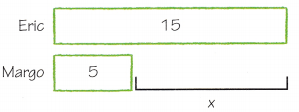Equation: _____________ x = ____________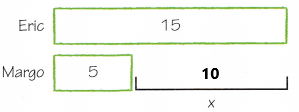Equation:
5 + x = 15
5 + 10 = 15
x = 10
Eric jogged 10 more laps than Margo.
Explanation:
Eric jogged 15 laps around a track. Margo jogged 5 laps. The equation is 5 + x = 15. The value of x is 10. So Eric jogged 10 more laps than Margo.

Question 3.
Sadie hit 17 golf balls from her bucket. Then there were 33 golf balls left in the bucket. How many golf balls did Sadie have in her bucket before she hit any golf balls?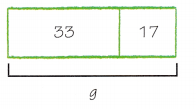Equation: ______________ g = ____________Equation:
33 + 17 = g
50 = g
Sadie have 50 golf balls in her bucket before she hit any golf balls.
Explanation:
Sadie hit 17 golf balls from her bucket. Then there were 33 golf balls left in the bucket. Add 33golf balls with 17 golf balls the sum is 50 golf balls. Sadie have 50 golf balls in her bucket before she hit any golf balls.

Write an equation. Then solve. (TEKS 5.4.B)

Question 4.
A store sells 8 small, 18 medium, and 20 large sweatshirts. Each sweatshirt costs $16. How much money, m, is the store paid for all the sweatshirts they sell? Answer:$16 x ( 8 + 18 + 20) = m
$16 x 46 = m$736 = m
The store paid $736 for all the sweatshirts they sold. Explanation: A store sells 8 small, 18 medium, and 20 large sweatshirts. Each sweatshirt costs$16. The equation is $16 x (8 + 18 + 20). First add 8 with 18 and 20 the sum is 46. Next multiply$16 with 46 the product is $736. The store paid$736 for all the sweatshirts they sold.

Question 5.
A caterer makes sandwiches from 8 loaves of bread. Each loaf makes 10 sandwiches. The caterer puts 3 ounces of turkey on each sandwich. How many ounces, z, of turkey does the caterer use?
8 x 10 = 80
80 x 3 = 240
The caterer used 240 ounces of turkey.
Explanation:
A caterer makes sandwiches from 8 loaves of bread. Each loaf makes 10 sandwiches. Multiply 8 loaves with 10 sandwiches the product is 80. The caterer puts 3 ounces of turkey on each sandwich. Multiply 3 ounces with 80 sandwiches the product is 240 ounces. The caterer used 240 ounces of turkey.

Question 6.
A train has 34 passengers in each of 3 cars and 28 passengers in each of 4 cars. How many passengers, p, are on the train?
Equation:
34 x 3 + 28 x 4 = p
102 + 112 = p
214 = p
There are 214 passengers on the train.
Explanation:
A train has 34 passengers in each of 3 cars and 28 passengers in each of 4 cars. The equation is 34 x 3 + 28 x 4 = p. Here p represents the number of passengers on the train. Multiply 34 with 3 the product is 102. Multiply 28 with 4 the product is 112. Add 102 with 112 the sum is 214. There are 214 passengers on the train.

Question 7.
There are 252 students at a school party. They are seated in groups of 9. Each group gets 3 bags of popcorn. How many bags of popcorn, p, are used for the party?
Equation:
(252 ÷ 9) x 3 = p
28 x 3 = p
84 = p
They used 84 bags of popcorn for the party.
Explanation:
There are 252 students at a school party. They are seated in groups of 9. Each group gets 3 bags of popcorn. The equation is (252 ÷ 9) x 3 = p. First divide 252 by 9 the result is 28. Multiply 28 with 3 the product is 84. They used 84 bags of popcorn for the party.

Question 8.
Ryan has 5 boxes with 8 markers in each box. He has 7 boxes with 10 markers in each box. He gives his friend one box of 8 markers and one box of 10 markers. Which equation can be used to find the total number of markers, m, that Ryan has left? (TEKS 5.4.B)
(A) 7 × 10 – 5 × 8 – 8 – 10 = m
(B) 8 – 5 × 8 + 10 – 7 × 10 = m
(C) 5 × 8 ÷ 7 × 10 – 8 – 10 = m
(D) 5 × 8 + 7 × 10 – 8 – 10 = m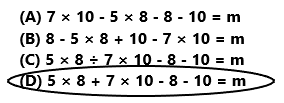Equation:
5 x 8 + 7 x 10 – 8 – 10 = m
40 + 70 – 8 – 10 = m
92 = m
The total number of markers that Ryan has left are 92.
So, option D is correct.
Explanation:
Ryan has 5 boxes with 8 markers in each box. Multiply 5 with 8 the product is 40. He has 7 boxes with 10 markers in each box. Multiply 7 with 10 the product is 70. He gives his friend one box of 8 markers and one box of 10 markers. The equation is 5 x 8 + 7 x 10 – 8 – 10 = m. Subtract 18 from 110 the difference is 92. The total number of markers that Ryan has left are 92. So, option D is correct.

Question 9.
Thora had $5 left in her pocket after spending$12 on snacks and souvenirs on a class trip. How much money did Thora take on the class trip? The equation t – 12 = 5 can be used to find t, the amount of money Thora took on the class trip. (TEKS 5.4)
(A) $17 (B)$14
(C) $7 (D)$16Equation:
t – $12 =$5
$17 –$12 = $5 t =$17
The amount of money Thora took on the class trip is $17. So, option A is correct. Explanation: Thora had$5 left in her pocket after spending $12 on snacks and souvenirs on a class trip. The equation is t – 12 = 5 can be used to find t, Here t is the amount of money Thora took on the class trip. Add$5 with $12 the sum is$17. The value of t is $17. So option A is correct. Question 10. Students earned$368 selling c, calendars. They earned $456 selling m, mugs. The price of each mug and each calendar is$8. The equation c = 368 ÷ 8 represents the number of calendars they sold. The equation m = 456 ÷ 8 represents the number of mugs they sold. How many more mugs than calendars did students sell? (TEKS 5.4.B)
(A) 57
(B) 103
(C) 11
(D) 46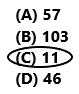Students earned $368 selling c, calendars. Equation: c = 368 ÷ 8 c = 46 Students sold 46 calendars. Students earned$456 selling m, mugs.
Equation:
m = 456 ÷ 8
m = 57
Students sold 57 mugs.
57 mugs – 46 calendars = 11 mugs
Students sold 11 more mugs than calendars.
So, option C is correct.
Explanation:
Students earned $368 selling c, calendars. They earned$456 selling m, mugs. The price of each mug and each calendar is \$8. The equation c = 368 ÷ 8 represents the number of calendars they sold. They sold 46 calendars. The equation m = 456 ÷ 8 represents the number of mugs they sold. They sold 57 mugs. Subtract 46 calendars from 57 mugs the difference is 11 mugs. Students sold 11 more mugs than calendars. So, option C is correct.

Question 11.
There are 180 students at summer camp. Each cabin houses 12 students. There are 2 counselors for each cabin. Solve the equation c = (180 ÷ 12) × 2 to find the number of c, counselors. (TEKS 5.4.B)Record your answer and fill in the bubbles on the grid. Be sure to use the correct place value.